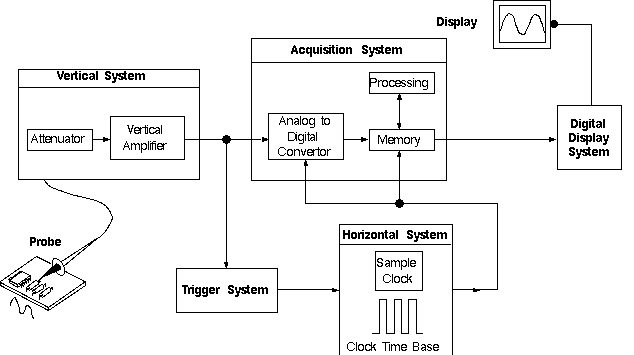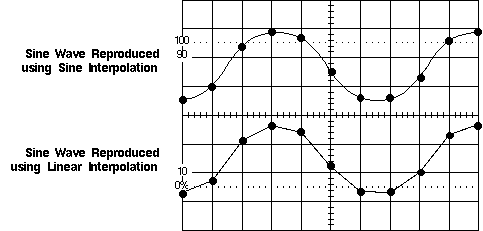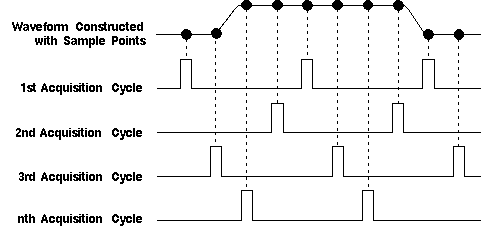Home > Electronic Tutorials > Oscilloscope Tutorial > How Does an Oscilloscope Work?

Online Oscilloscope Tutorial

# About OSCILLOSCOPE - How Does an Oscilloscope Work?

To better understand the oscilloscope controls, you need to know a little more about how oscilloscopes display a signal. Analog oscilloscopes work somewhat differently than digital oscilloscopes. However, several of the internal systems are similar. Analog oscilloscopes are somewhat simpler in concept and are described first, followed by a description of digital oscilloscopes.

Analog Oscilloscopes

When you connect an oscilloscope probe to a circuit, the voltage signal travels through the probe to the vertical system of the oscilloscope. Following Figure is a simple block diagram that shows how an analog oscilloscope displays a measured signal.Analog Oscilloscope Block Diagram

Depending on how you set the vertical scale (volts/div control), an attenuator reduces the signal voltage or an amplifier increases the signal voltage.

Next, the signal travels directly to the vertical deflection plates of the cathode ray tube (CRT). Voltage applied to these deflection plates causes a glowing dot to move. (An electron beam hitting phosphor inside the CRT creates the glowing dot.) A positive voltage causes the dot to move up while a negative voltage causes the dot to move down.

The signal also travels to the trigger system to start or trigger a "horizontal sweep." Horizontal sweep is a term referring to the action of the horizontal system causing the glowing dot to move across the screen. Triggering the horizontal system causes the horizontal time base to move the glowing dot across the screen from left to right within a specific time interval. Many sweeps in rapid sequence cause the movement of the glowing dot to blend into a solid line. At higher speeds, the dot may sweep across the screen up to 500,000 times each second.

Together, the horizontal sweeping action and the vertical deflection action traces a graph of the signal on the screen. The trigger is necessary to stabilize a repeating signal. It ensures that the sweep begins at the same point of a repeating signal, resulting in a clear picture as shown in following figure.Triggering Stabilizes a Repeating Waveform

In conclusion, to use an analog oscilloscope, you need to adjust three basic settings to accommodate an incoming signal:

• The attenuation or amplification of the signal. Use the volts/div control to adjust the amplitude of the signal before it is applied to the vertical deflection plates.
• The time base. Use the sec/div control to set the amount of time per division represented horizontally across the screen.
• The triggering of the oscilloscope. Use the trigger level to stabilize a repeating signal, as well as triggering on a single event.

Also, adjusting the focus and intensity controls enables you to create a sharp, visible display.

Digital Oscilloscopes

Some of the systems that make up digital oscilloscopes are the same as those in analog oscilloscopes; however, digital oscilloscopes contain additional data processing systems. With the added systems, the digital oscilloscope collects data for the entire waveform and then displays it.

When you attach a digital oscilloscope probe to a circuit, the vertical system adjusts the amplitude of the signal, just as in the analog oscilloscope.

Next, the analog-to-digital converter (ADC) in the acquisition system samples the signal at discrete points in time and converts the signal's voltage at these points to digital values called sample points. The horizontal system's sample clock determines how often the ADC takes a sample. The rate at which the clock "ticks" is called the sample rate and is measured in samples per second.

The sample points from the ADC are stored in memory as waveform points. More than one sample point may make up one waveform point.

Together, the waveform points make up one waveform record. The number of waveform points used to make a waveform record is called the record length. The trigger system determines the start and stop points of the record. The display receives these record points after being stored in memory.

Depending on the capabilities of your oscilloscope, additional processing of the sample points may take place, enhancing the display. Pretrigger may be available, allowing you to see events before the trigger point.Digital Oscilloscope Block Diagram

Fundamentally, with a digital oscilloscope as with an analog oscilloscope, you need to adjust the vertical, horizontal, and trigger settings to take a measurement.

Sampling Methods

The sampling method tells the digital oscilloscope how to collect sample points. For slowly changing signals, a digital oscilloscope easily collects more than enough sample points to construct an accurate picture. However, for faster signals, (how fast depends on the oscilloscope's maximum sample rate) the oscilloscope cannot collect enough samples. The digital oscilloscope can do two things:

• It can collect a few sample points of the signal in a single pass (in real-time sampling mode) and then use interpolation. Interpolation is a processing technique to estimate what the waveform looks like based on a few points.
• It can build a picture of the waveform over time, as long as the signal repeats itself (equivalent-time sampling mode).

Real-Time Sampling with Interpolation

Digital oscilloscopes use real-time sampling as the standard sampling method. In real-time sampling, the oscilloscope collects as many samples as it can as the signal occurs. See following figure for single-shot or transient signals you must use real time sampling.Real Time Sampling Diagram

Digital oscilloscopes use interpolation to display signals that are so fast that the oscilloscope can only collect a few sample points. Interpolation "connects the dots."

Linear interpolation simply connects sample points with straight lines. Sine interpolation (or sin x over x interpolation) connects sample points with curves. (See Following Figure) Sin x over x interpolation is a mathematical process similar to the "oversampling" used in compact disc players. With sine interpolation, points are calculated to fill in the time between the real samples. Using this process, a signal that is sampled only a few times in each cycle can be accurately displayed or, in the case of the compact disc player, accurately played back.Linear and Sine Interpolation Diagram

Equivalent-Time Sampling

Some digital oscilloscopes can use equivalent-time sampling to capture very fast repeating signals. Equivalent-time sampling constructs a picture of a repetitive signal by capturing a little bit of information from each repetition. (See Following Figure) You see the waveform slowly build up like a string of lights going on one-by-one. With sequential sampling the points appear from left to right in sequence; with random sampling the points appear randomly along the waveform.Equivalent-time Sampling Diagram

Note: To report broken links or to submit your projects please send email to Webmaster

 Discover